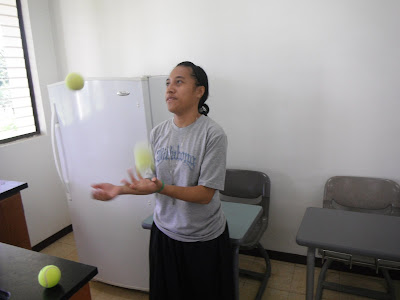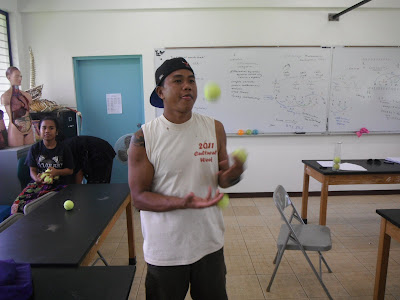### Site Swap Laboratory

Laboratory fifteen in physical science sought to push the boundaries on the mathematical box for the students. In laboratory one a quote from Freeman Dyson was used to start a journey through the mathematical models that explain physical systems. Dyson calculated how an electron ought to behave. Later someone went into a laboratory and the electron behaved as predicted by the mathematical model.Maria-Paul

The relationships described above are algebraic mathematical models. Much of the mathematics curriculum is centered on algebra in part because algebra is important to describing the physical world. There are, however, other mathematical models, non-algebraic models. This laboratory seeks to broaden the students mathematical horizons by introducing a mathematical model and notation that is not algebraic. In laboratory fifteen the students were introduced to the mathematics of site swaps.Darla

In an attempt to connect site swap theory back to the language of algebraic equations, after introducing site swap notation I referred to sequences such as 33342333 as site swap equations. The sequence is a mathematical statement that can be true (juggable) or false (not juggable).Jamori

This laboratory continues to provide a fun way to wrap up a term of exploring the mathematics at the core of physical science while expanding the students thinking with a mathematics system like nothing they have ever seen before.Reliann

The laboratory is also an end of term enjoyable experience. As the course is not required by any major, the students are primarily from majors other than those in the natural sciences. For many of these students science is a requirement, possibly even a dreaded requirementI want the students to have the chance to do science, engage in exploring systems, grapple with the mathematical language underneath physical science. I can only hope that the students catch a glimpse of the beauty of science - of even pure science for science's sake.Sepe opted out

I want the students to have good memories of the course, to think positively of science and of their own ability to do science. Thus I try to end the course on George M. Cohan's advice to "Always leave them laughing when you say goodbye."Jessica CurleyRicksonMehrun OttoBeverly Ilemangilish juggles four balls using multiplex catching of varying pairs of balls.BeverlyBeverlyDarby, Darla's twin sisterMisako and LarryDarby